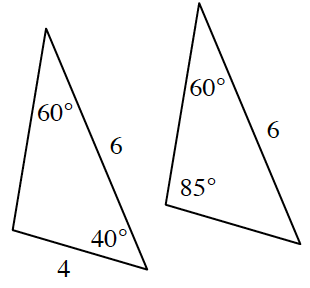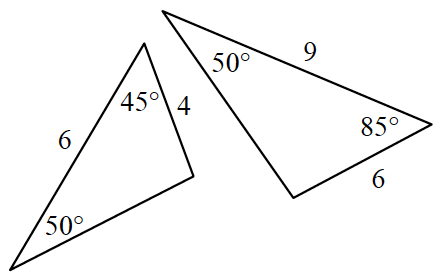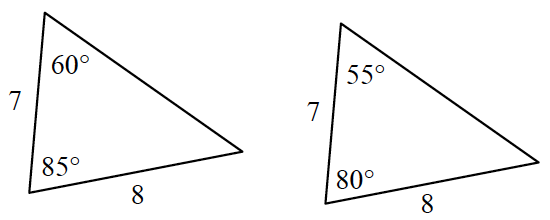### Home > GC > Chapter 3 > Lesson 3.2.4 > Problem3-80

3-80.

Determine which of the following pairs of triangles are similar. Explain your work.

1.Find the missing angle measure for each triangle to see if $\text{AA}\sim$ applies.

The triangles are not similar because they have different angle measures.

1.Find the missing angle measure for each triangle to see if $\text{AA}\sim$ applies.

1.• Write a ratio for each pair of sides. If the triangles are similar, all three ratios should be equal.

Is this true?